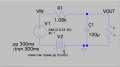# Ltspice Help- Analysing Load current of Capacitance multiplier and RC filter circuit??

#### Himanshoo

Joined Apr 3, 2015
265
Hi friends,

I have simulated two circuits, one is an RC filter and other is a Capacitance multiplier.
My aim of experiment is to validate a decrease in Iout (load current) of CM circuit as compared to load current in RC filter circuit.

Theoretically,
Iout (CM circuit) < Iout (RC filter)
Vp-p (CM circuit) < Vp-p (RC filter)

My results:
1.RC filter

Vout (dc) = 0.0458716 ( .op 300ms)
Iout (dc) = 0.00458716

Vout (ac) = 43.7 ~ 48 mV (.tran 300ms)
Iout (ac) = 4.80 ~ 4.37 mA

Vp-p (ripple) = 0.00437018 (.meas)

2.CM circuit

Vout (dc) = 0.323751
Iout (dc) = 0.0323751
Vout (ac) = 322 ~ 327 mV
Iout (ac) = 32.65 ~ 32.15 ma

Vp-p (ripple) = 0.00521016 (.meas)

From the above results its quite clear that implementing CM circuit causes Iout to increase which particularly against the aim of the CM circuit. As the CM circuit works by decreasing the load current which according to load POV can be visualised as increase in capacitance.
We can also see the peak to peak ripple voltage in both the circuits, Vp-p for CM circuit should be less than Vp-p ripple of an RC filter, but again the simulations results are quite opposite.

Whats is the error ??#### Attachments

• 1.8 KB Views: 3
• 1,014 bytes Views: 0

#### Ian0

Joined Aug 7, 2020
8,426
In the RC filter, R1 is limiting the current. Your output load of 10Ω, is in a potential divider with R1, which gives an output voltage of R2/(R1+R2) * Vin = 45mV
In the transistor circuit the output voltage is the R1:R3 potential divider less the Vbe of the transistor.
= 1.19V-0.6V = 0.59V
Higher output voltage gives higher output current into the same load.